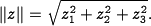# FAQ: What do the double vertical bars mean at the end of Section 4.1?The expression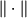represents the magnitude of the vector enclosed between the sets of double vertical bars. For example, if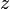is a vector of length three with elements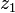,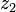,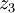, then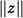is given by